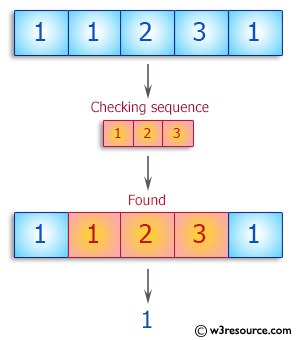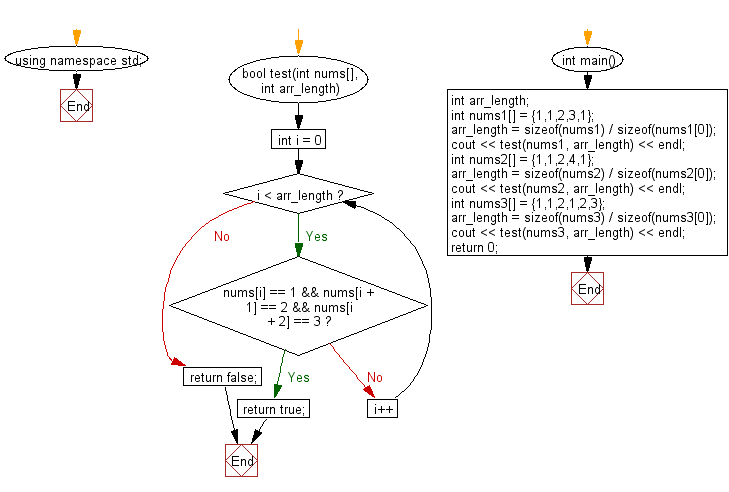﻿ C++ : Check whether a sequence appears in a array somewhere# C++ Exercises: Check whether the sequence of numbers 1, 2, 3 appears in a given array of integers somewhere

## C++ Basic Algorithm: Exercise-31 with Solution

Write a C++ program to check whether the sequence of numbers 1, 2, 3 appears in a given array of integers somewhere.

Sample Solution:

C++ Code :

``````#include <iostream>

using namespace std;

bool test(int nums[], int arr_length)
{

for (int i = 0; i < arr_length; i++)
{
if (nums[i] == 1 && nums[i + 1] == 2 && nums[i + 2] == 3)
return true;
}
return false;
}

int main()
{
int arr_length;
int nums1[] = {1,1,2,3,1};
arr_length = sizeof(nums1) / sizeof(nums1);
cout << test(nums1, arr_length) << endl;
int nums2[] = {1,1,2,4,1};
arr_length = sizeof(nums2) / sizeof(nums2);
cout << test(nums2, arr_length) << endl;
int nums3[] = {1,1,2,1,2,3};
arr_length = sizeof(nums3) / sizeof(nums3);
cout << test(nums3, arr_length) << endl;
return 0;
}
``````

Sample Output:

```1
0
1
```

Pictorial Presentation:Flowchart:C++ Code Editor:

Contribute your code and comments through Disqus.

What is the difficulty level of this exercise?

﻿